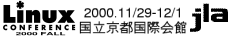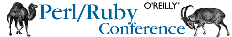\$B%H%C%W%Z!]%8(B \$B%\$%Y%s%H35MW(B \$B\$40';"(B \$B%W%m%0%i%`(B \$B4pD49V1i(B \$B%A%e!]%H%j%"%k(B \$B%9%Z%7%c%k%;%C%7%g%s(B \$B%+%s%U%!%l%s%9(B \$B!!(B\$B!&(BLinux \$B!!(B\$B!&(BPerl/Ruby \$B%o!<%/%7%g%C%W(B, BOF \$B%W%m%0%i%`%F!<%V%k(B 11\$B7n(B29\$BF|(B(\$B?e(B) 11\$B7n(B30\$BF|(B(\$BLZ(B) 12\$B7n(B1\$BF|(B(\$B6b(B) \$BF@!9>pJs(B \$B;22C?=\$79~\$_(B \$BNA6bBN7O(B \$B=IGq;\@_0FFb!&M=Ls(B \$B?=9~\$K4X\$9\$k(B FAQ \$B2q>l0FFb(B \$B=PE80FFb(B \$B%9%]%s%5!<(B \$B \$B%9%T!<%+!<>R2p(B \$B \$B2a5n\$N(BLC LC2000 Spring Linux Conference '99 Linux Conference '98

# \$B4pD49V1i(B

\$B\$^\$D\$b\$H\$f\$-\$R\$m(B

Ruby\$B\$,\$I\$3\$+\$iMh\$F!"\$J\$s\$N\$?\$a\$K\$3\$3\$K\$"\$j!"\$=\$7\$F\$3\$3\$+\$i\$I\$3\$X9T\$/(B \$B\$N\$+!#(BRuby\$B\$NNr;K!";WA[!"@_7W86M}\$d<~JU;v>p\$+\$i!":#8e\$NM=Dj\$dL\$Mh\$N;Q(B \$B\$K;j\$k\$^\$G!"(BRuby\$B\$N\$"\$l\$3\$l\$r8l\$j\$^\$7\$g\$&!#(B

(11/30 13:30\$B!A(B 90 \$BJ,(B)Eric Steven Raymond
Linux\$B\$NBfF,\$r7@5!\$H\$7\$FCmL\\$r=8\$a\$F\$\$\$k%=%U%H%&%'%"3+H/-Mh\$N(B \$BE8K>\$K\$D\$\$\$F8l\$k!#(B
(12/1 12:30\$B!A(B 40\$BJ,(B)Bruce Momjian
(12/1 10:30\$B!A(B 90 \$BJ,(B)

\$B\$3\$N%5%\$%H\$K4X\$9\$k\$40U8+!&\$4MWK>\$O(B lc-info@linux.or.jp \$B\$^\$G\$*4j\$\$CW\$7\$^\$9(B.